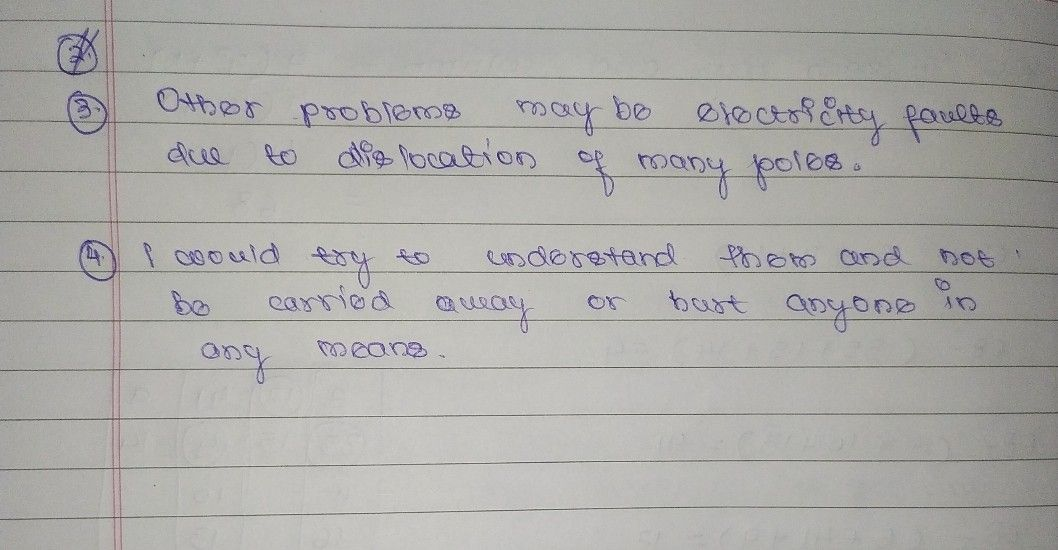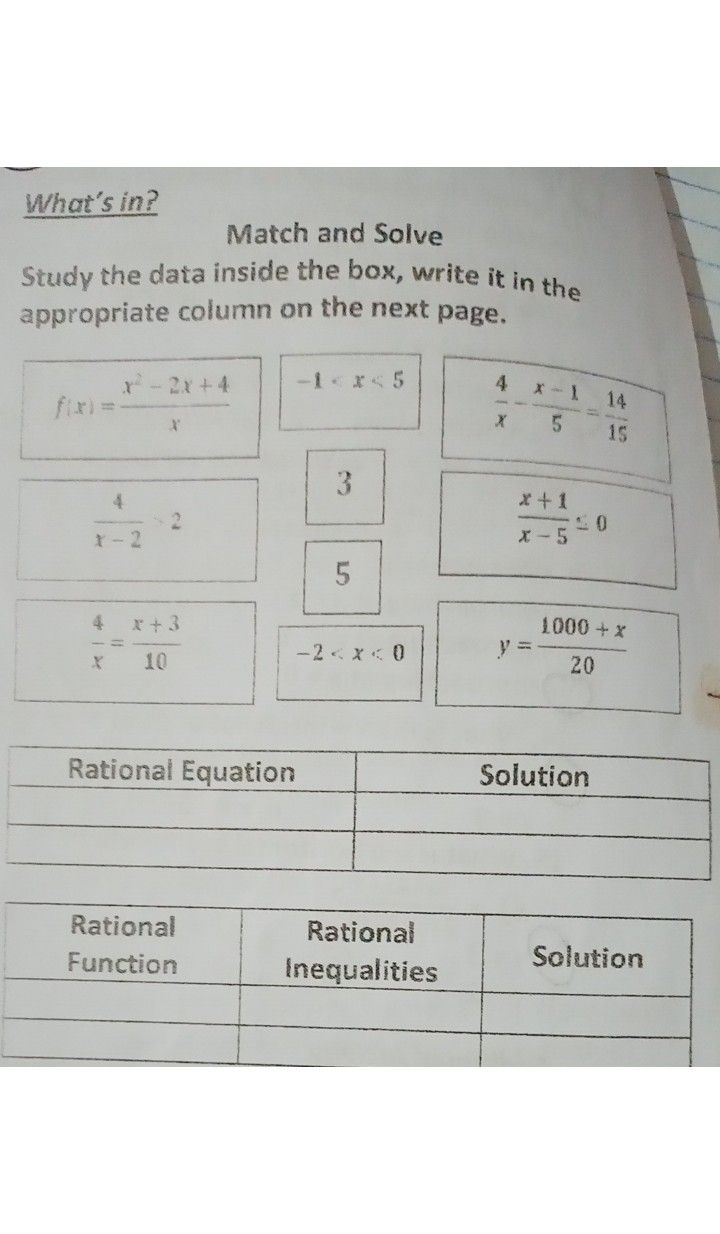Symbol
Problem1. Suppose the officials conducted a survey for 4 days to determine the actual number of families residing in the barangay and the secretary constructed a table to keep track of the data. Complete the table $below$ Day No. of Families Amt recalved per family 0. $850$ P520.00 1 $855$ $f5-1\left(o9\left(2$ 2 $882$ 3 $910$ P $8$ $71$ 4 $933$ $474-76$ $2.$ Create a model $or$ equation that will represent to the amount of relief each family will recelve bearing in mind that the number of $am=5$ will $≠\left(x\right)442$ 000 vary.E 3. After resolving the amount of relief each family may receive, what other problem may arise in the current situation? $4$ If you are one of the residents of that barangay what $lwi1$ $be$ your participation to help the $oficials7$
10th-13th grade
Calculus
Search count: 107
SolutionQanda teacher - Shewtaplease ask if any doubtsStudent